## Example Questions

← Previous 1

### Example Question #1 : Rational Expressions

Simplify the expression.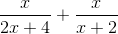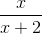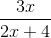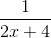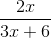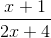Explanation:

To add rational expressions, first find the least common denominator. Because the denominator of the first fraction factors to 2(x+2), it is clear that this is the common denominator. Therefore, multiply the numerator and denominator of the second fraction by 2.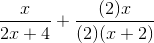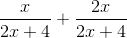This is the most simplified version of the rational expression.

### Example Question #2 : Rational Expressions

Simplify the following: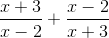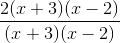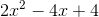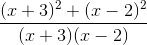Explanation:

To simplify the following, a common denominator must be achieved. In this case, the first term must be multiplied by (x+2) in both the numerator and denominator and likewise with the second term with (x-3).### Example Question #1 : How To Evaluate Rational Expressions

If √(ab) = 8, and a= b, what is a?

10

16

4

64

2

4

Explanation:

If we plug in a2 for b in the radical expression, we get √(a3) = 8. This can be rewritten as a3/2 = 8. Thus, loga 8 = 3/2. Plugging in the answer choices gives 4 as the correct answer.

### Example Question #4 : Rational Expressions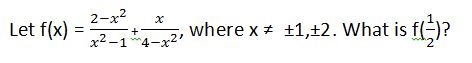–11/5

–37/15

9/5

37/15

–9/5

–11/5

Explanation: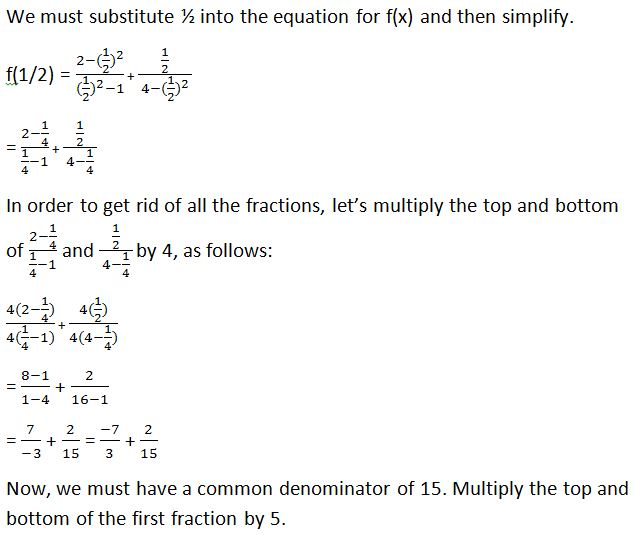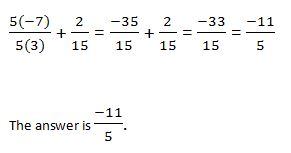### Example Question #5 : Rational Expressions

If Jill walks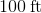in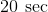, how long will it take Jill to walk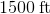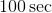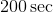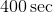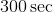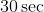Explanation:

To solve this, we need to set a proportion.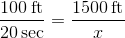Cross Multiply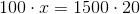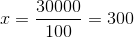So it will take Jillto walk### Example Question #6 : Rational Expressions

If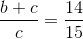, then which of the following must be also true?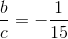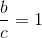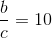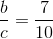Explanation: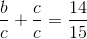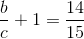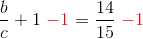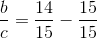### Example Question #2401 : Act Math

Which of the following is equivalent to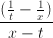? Assume that denominators are always nonzero.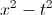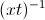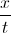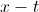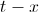Explanation:

We will need to simplify the expression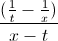. We can think of this as a large fraction with a numerator of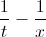and a denominator of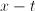.

In order to simplify the numerator, we will need to combine the two fractions. When adding or subtracting fractions, we must have a common denominator.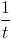has a denominator of, and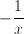has a denominator of. The least common denominator that these two fractions have in common is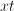. Thus, we are going to write equivalent fractions with denominators of.

In order to convert the fraction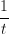to a denominator with, we will need to multiply the top and bottom by.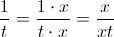Similarly, we will multiply the top and bottom ofby.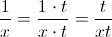We can now rewriteas follows:=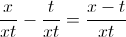Let's go back to the original fraction. We will now rewrite the numerator:=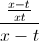To simplify this further, we can think ofas the same as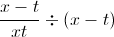. When we divide a fraction by another quantity, this is the same as multiplying the fraction by the reciprocal of that quantity. In other words,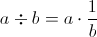.=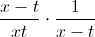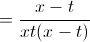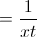Lastly, we will use the property of exponents which states that, in general,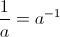.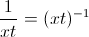The answer is.

### Example Question #1 : How To Multiply Rational Expressions

Simplify (4x)/(x– 4) * (x + 2)/(x– 2x)

4/(x – 2)2

(4x+ 8x)/(x+ 8x)

4/(x + 2)2

x/(x + 2)

x/(x – 2)2

4/(x – 2)2

Explanation:

Factor first.  The numerators will not factor, but the first denominator factors to (x – 2)(x + 2) and the second denomintaor factors to x(x – 2).  Multiplying fractions does not require common denominators, so now look for common factors to divide out.  There is a factor of x and a factor of (x + 2) that both divide out, leaving 4 in the numerator and two factors of (x – 2) in the denominator.

### Example Question #9 : Rational Expressions

what is 6/8 X 20/3

18/160
5
9/40
120/11
3/20
Explanation:

6/8 X 20/3 first step is to reduce 6/8 -> 3/4 (Divide top and bottom by 2)

3/4 X 20/3 (cross-cancel the threes and the 20 reduces to 5 and the 4 reduces to 1)

1/1 X 5/1 = 5

### Example Question #10 : Rational Expressions

Evaluate and simplify the following product: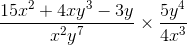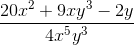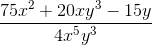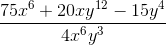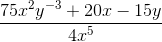Explanation:

The procedure for multplying together two rational expressions is the same as multiplying together any two fractions: find the product of the numerators and the product of the denominators separately, and then simplify the resulting quotient as far as possible, as shown: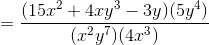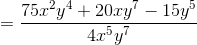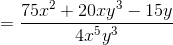← Previous 1